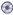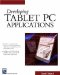# Math and Random Number Functions in VB .NETDownload CD Content

In previous versions of VB, there were a multitude of functions that aided a developer in the creation of complex mathematical development. These functions also exist in VB .NET, but like everything else, they are implemented a little differently and reside in the System.Math class.

## The SYSTEM MATH Class

The System.Math class contains a variety of methods that you can use for mathematical calculations. There are functions such as square root, pi, absolute value, rounding, and trigonometry to name a few. We look at each of them with a little detail here, and later in the book, we use them to build a fully functional calculator.

### Raising to a Power

We begin our discussion of the System.Math class by looking at the Pow method. The Pow method is used to raise a number to a power. For example, you can raise a number to a power of 2 with the following code:

```Dim X As Integer
X = Math.Pow(2, 2)```

The previous code is assuming that we imported the System.Math class. We then assign the integer variable X equal to 2 to a power of 2. We can also rewrite the code as follows:

```Dim X As Integer
X = 2 ^ 2```

Now, let's use the Pow method to perform a useful calculation. For example, suppose we are interested in calculating the area of a circle (it is equal to pi * radius squared). We can do this as follows:

```Dim X as Double

```

The previous code assigns a value of 50 to the radius and then calculates the area and assigns it to X. You'll notice that we used Math.PI for the value of pi. It is another built-in method available to us in VB .NET.

### Square Root

The square root method (Sqrt), like the other VB .NET math functions, resides in the System.Math class. You can use it to calculate the square root of a value. The following example demonstrates its use:

```Dim X as Double
X = Sqrt (100)```

### Absolute Value

We can use the absolute value (Abs) method to return an absolute value of a number. If you are unfamiliar with the absolute value, it is simply the value of a number, without regard to its sign. In other words, it is a positive number. Here is an example:

```Dim X as Double

X = Abs( -10.5)```

This returns a value of 10.5. Similarly, the following example returns the same value:

```Dim X as Double

X = Abs(10.5)```

However, if you want to return the sign of a number, you can use the Sign method.

If the number is negative, Sign returns -1; if it's positive, Sign returns 1; and if the number is equal to 0, Sign returns 0. Here is an example:

```Dim X1, X2, X3 As Double
Dim X4 As Integer
X1 = 5
X2 = -5
X3 = 0
X4 = Sign(X1)```

This returns a value of 1.

```Dim X1, X2, X3 As Double
Dim X4 As Integer
X1 = 5
X2 = -5
X3 = 0
X4 = Sign(X2)```

This returns a value of -1.

```Dim X1, X2, X3 As Double
Dim X4 As Integer
X1 = 5
X2 = -5
X3 = 0
X4 = Sign(X3)```

This returns a value of 0.

### Rounding Numbers

If you need to round values to the nearest integer value, you can use the Round method. As an example, suppose you have a value of 5.12345 and need to round it:

```Dim X as Double
X = Math.Round(5.12345)```

This rounds it to 5. There are a few things to remember when using the Round method. If you have a number that is between two numbers (such as 5.5 or 6.5), the method may not return what you would at first believe. Most people would round these values up to the next highest value; however, if you create the following code, something interesting happens:

```Dim X1, X2 As Double
X1 = Math.Round(5.5)
X2 = Math.Round(6.5)```

The values of X1 and X2 both return values of 6. This is because the Round method actually returns the EVEN number closest to the two. To truncate a number in VB .NET, you can use the Floor method. It returns the largest whole number smaller than the orginal number. So, the following code returns 5:

```Dim X as Double
X = Math.Floor(5.6)```

Negative values can behave unexpectedly, so you have to pay special attention to them. For example, if you create a similar project with a negative value, it returns a -6:

```Dim X as Double
X = Math.Floor(-5.6)
```

### Trigonometry

The Math class also contains a number of methods for making trigonometric and logarithmic calculations. These include methods for Sin, Cos, Tan, and Atn. They work like the other methods we have been looking at throughout this chapter:

```Dim X as Double
X = Sin(1.1)```

This returns a value in radians. If you want to convert from radians to degrees, you multiply by 180/pi:

```Dim X As Double
X = 180 / Math.PI * Sin(1.1)
Debug.WriteLine(X)```
 Note If you have a degree value, you can convert it to radians by multiplying by pi/180.

### Logarithms

The System.Math class also provides functionality for logs and natural logs. You can use the Exp method of the class to return e raised to a power:

```Dim X as Double
X = Math.Exp(2)```

You can also use the Log method of the Math class to return the natural logarithm of a number:

```Dim X as Double
X = Math.Log(5)```

## Creating Your Own Math Functions

There are obviously many great methods related to math built into the System.Math class. However, there are going to be times when you need to create your own. We can take a simple example for conversions to see how and why you will do so. For example, let's suppose you are trying to convert Celsius to Fahrenheit. The formulas for conversion are as follows:

Celsius to Farhenheit: Value * 1.8 + 32

Farhenheit to Celsuis: (Value - 32)/1.8

The functions can then be created as follows:

```Function CToF(ByVal value as Single) as Single
CToF = value * 1.8 +32
End Function

Function FToC(ByVal value as Single) as Single
FToC=(value-32)/1.8
End Function```

We can use the functions in a program as follows:

```Dim X As Single
X = CToF(25)```

As an additional example, suppose we need to return the decimal part of a number. We can calculate this simply by subtracting the number from the decimal portion:

```Function Decm(Value as Double) As Double
Decm = value - fix(value)
End Function```

We could then use this as follows:

```Dim X as Double
X = Decm(10.5)```

This returns a value of 0.5.

### Generating Random Numbers

The System.Random class is used to draw a random number, and unlike the VB6 Rnd function, System.Random can return both decimal and whole random numbers. Additionally, unlike Rnd, System.Random automatically seeds its random number generator with a random value derived from the current date and time.

You can use the NextDouble method of System.Random to return a Double random number between 0 and 1. You can use the Next method to return an Integer random number between two integer values.

```Dim X As Double
Dim rnd As System.Random = New System.Random()
Dim i As Integer

For i = 1 To 10
X = Round(rnd.NextDouble() * 10)
Debug.WriteLine(X)
Next```

This code displays a series of random numbers between 0 and 10.

You can also use the Next method as follows:

```Dim X As Double
Dim rnd As System.Random = New System.Random()
Dim i As Integer

For i = 1 To 10
X = rnd.Next(0, 10)
Debug.WriteLine(X)
Next```

## Summary

In this chapter, we worked on several topics that are related to the math functions available in VB .NET. This is the final chapter that consists of only text information. In most of the remaining chapters, we build applications. In doing so, we put some of the information that we have already learned to good use and also learn about many new topics. In Chapter 9, Your First Program, we create our first VB .NET application.Developing Tablet PC Applications (Charles River Media Programming)
ISBN: 1584502525
EAN: 2147483647
Year: 2003
Pages: 191